Industry Article

# Efficient Orthogonal Variable Optimization Algorithm for Communication Systems

## This article discusses an algorithm to find the optimum adjusted point in a two-dimensional space with orthogonal input vectors. The algorithm solves equations for intersecting circles based on measured data points.

Several key system performance metrics are determined by orthogonal input parameters corresponding to amplitude and phase; two examples are quadrature modulator carrier feedthrough and sideband suppression. These parameters are improved by optimizing DC offset balance and the amplitude and phase balance between the quadrature baseband inputs of the modulator.

Because finding the optimal adjustment of these parameters is not a trivial task given the two-dimensional space, in this article I will discuss a Python algorithm to find the optimal adjusted point in a two-dimensional space with orthogonal input vectors. The algorithm solves equations for intersecting circles based on measured data points and finds the optimal point in as few as four iterations. To exemplify the technique, I used the Texas Instruments (TI) AFE7070 integrated digital-to-analog converter (DAC) plus the quadrature modulator carrier feedthrough parameter.

### Optimizing Spurious Emissions

Communication systems strive to minimize spurious emissions. Some of these spurious emissions originate from orthogonal input signal mismatch. For example, DC offset mismatch and amplitude/phase imbalance will affect carrier feedthrough and sideband suppression parameters, respectively, in analog quadrature modulators. Adjusting the input variables to an optimal setpoint minimizes spurious output.

Figure 1 represents the measured parameter as the magnitude (r) of the vector from the optimized point, [x0,y0], to the actual point on a two-dimensional plane. The parameter value is bound by [0,1], a unit circle. A 0 represents complete cancellation or no signal and a 1 represents no cancellation or a full signal. In decibel scales, the function is bound by [-inf,0]. The circle of radius r represents all possible x,y points that achieve the same magnitude.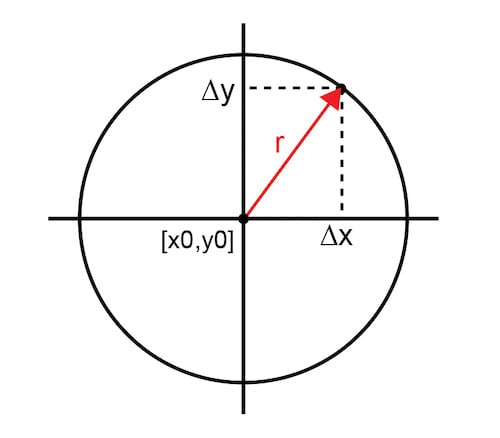##### Figure 1. Vector from optimized point to actual point

Equation 1 represents the function in its simplest form: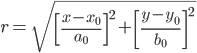(1)

Constants [a0,b0] represent system-dependent normalization factors to keep the maximum result less than 1. Technically, Equation 1 describes an ellipse, as the scaling factors for each independent variable do not need to be the same. For simplicity in this analysis, a0 is equal to b0 so that the curves are truly circles.

The goal is to find the optimal point [x0,y0] that minimizes the measurement parameter (r) as quickly as possible. Finding that point efficiently with statistically varying input parameters is challenging. The traditional hunt-and-peck approach uses successive trials to narrow down to the optimal point. Although this yields the desired solution, it takes too many iterations when convergence time is critical, necessitating a new method.

### Intersecting Circles

Ideally, exactly three measurement iterations using intersecting circles determine the optimal point. The first measured result at an arbitrary input point [x1,y1] defines infinite possibilities for the optimal point represented by circle A of radius r1. Adding a second data point yields another infinite data set represented by circle B; however, the intersections of the two circles narrow down the solution to two points. A third data point and corresponding circle C provide a third curve where there is only a single point of mutual intersection. That intersection point is the optimal point. Figure 2 shows the progression of intersecting circles that culminate in the definitive optimal point.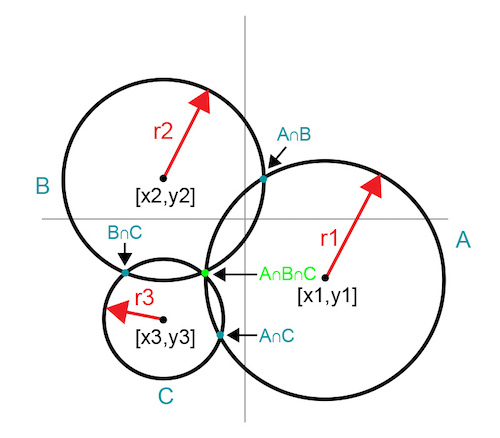##### Figure 2. Intersecting circles reveal a common intersection point

This technique reveals the optimal point in exactly three iterations. A fourth iteration measures the optimal point to confirm and document the result.

Figure 3 illustrates a technique to eliminate one iteration by using empirically known boundary information in the x-y plane. Select the initial point at one of the boundary points so that the first curve (A) is a 90-degree arc. Select the second point on the first arc by moving in either the x or y direction.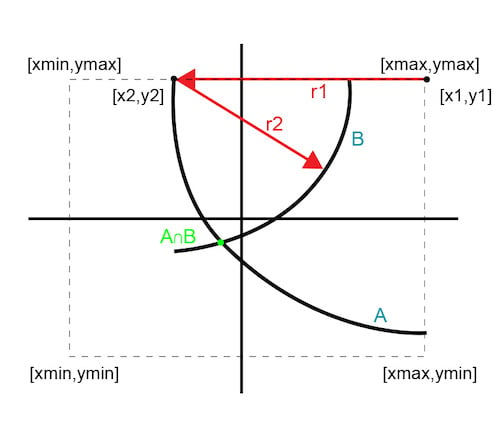##### Figure 3. Two-iteration solution

Since the second point is still on the boundary edge, its curve is a sub-180-degree arc. The intersection of these two curves provides a single optimal point. This approach reveals the optimal point in just two iterations and confirms it with three.

### Successive Circle Approximation

The optimal solution depends on the accuracy of the mathematical equation. In a real measurement situation, several assumptions or errors will impact the results. The measured signals are very small; noise and measurement tolerances introduce errors. Using perfect circles instead of ellipses introduces some uncertainty. The scaling factor assumptions needed to solve multiple equations also introduce uncertainty. These errors and assumptions translate to fuzziness in the curves.

Figure 4 illustrates how fuzzy curves do not guarantee an exact intersection point; instead, they define a convergence area.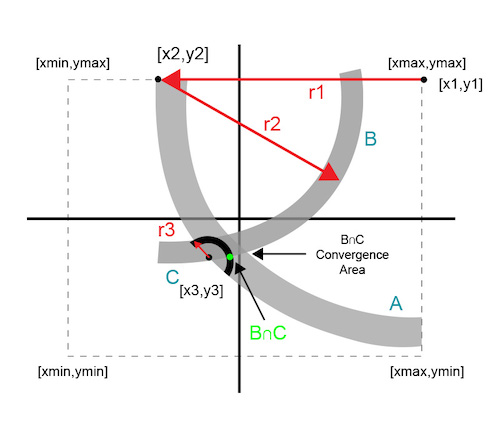##### Figure 4. Successive circle intersection approximation

Each additional data point uses the data from the previous iteration. Successive circles converge to a solution area that is within the system’s minimum threshold.

### Measurement Example

The example uses the AFE7070 DAC and optimizes for the carrier feedthrough parameter. The AFE7070 is a convenient platform, since the DAC and modulator are integrated. The DC offset imbalance on the modulator’s quadrature inputs determines the carrier feedthrough performance. The AFE7070 has an internal digital tuning feature to minutely control the DC offset balance. It does not take much; the resolution is on the scale of microvolts.

The x,y parameters for the example are integer digital step values that control the DC level on the quadrature inputs. Previous statistical sampling of the device provided the x,y bounds of the input variables as well as the step tables used in the calculations. The step tables provide a “conversion” of a measured carrier feedthrough in decibel milliwatts to a delta-x (or delta-y) factor.

A high (or in other words, poor) measured value means that the setting is way off and needs a larger delta to achieve the optimal point. Conversely, a low value means that the setpoint is close and needs a small correction. This bit of “homework” is necessary to ensure that initial guess points are not too far off, and to reduce the iteration time to the bare minimum.

Figure 5 shows the Python algorithm that finds the optimal input variables in four iterations or less.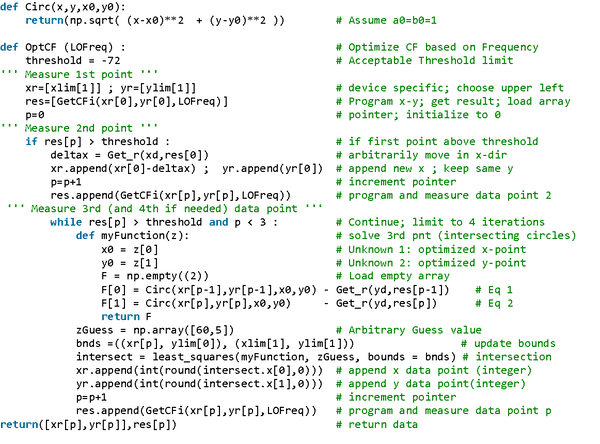##### Figure 5. Python optimization algorithm

The functions “Get_r” and “GetCFi” are device-specific measurements. I have omitted the code for brevity, as it is inconsequential for demonstrating the optimization algorithm. In your applications, these functions are pertinent to the programming and measurement of the device parameter in your system.

### Conclusion

For the AFE7070 DAC, in most cases, the algorithm optimizes carrier feedthrough within three iterations and in less than 1.7 s, gated primarily by the spectrum analyzer settling and sweep time. Previous step methods took nearly 20 iterations and more than 20 s to complete. This algorithm represents a speed improvement of over 10 times compared to the traditional approach. Other communication system parameters dependent on orthogonal input variables of gain and phase can also use this algorithm to efficiently home in on an optimal solution.

Industry Articles are a form of content that allows industry partners to share useful news, messages, and technology with All About Circuits readers in a way editorial content is not well suited to. All Industry Articles are subject to strict editorial guidelines with the intention of offering readers useful news, technical expertise, or stories. The viewpoints and opinions expressed in Industry Articles are those of the partner and not necessarily those of All About Circuits or its writers.